Your data analysis solution

# Gage R&R for quantitative data in Excel tutorial

2017-10-20

This tutorial will help you set up and interpret a Gage R&R (Reproducibility & Repeatability) analysis on quantitative data in Excel using the XLSTAT software.

## Dataset for Gage R&R: control and validate your measurement method and systems, when having several quantitative measures taken by one or more operators on several parts

An Excel sheet containing both the data and the results for use in this tutorial can be downloaded by clicking here.

The data are from [Montgomery, D.C. (2001), Introduction to Statistical Quality Control, 4th edition, John Wiley & Sons].

They correspond to a measurement system analysis with 10 parts, 3 operators and 3 repetitions for each measurement.

## Measurement System Analysis or Gage Repeatability and Reproducibility

Measurement System Analysis (MSA) or Gage R&R (Gage Repeatability and Reproducibility) is a method to control and judge a measurement process. It is useful to determine which sources are responsible for the variation of the measurement data. Variability can be caused by the measurement system, the operator or the parts. Gage R&R applied to quantitative measurements is based on two common methods: ANOVA and R control charts.

The word "gage" (or gauge) refers to the fact that the methodology is aimed at validating instruments or measurement methods.

A measurement is "repeatable" if the measures taken by a given operator for the same object (product, unit, part, or sample, depending of the field of application) repeatedly, do not vary above a given threshold. If the repeatability of a measurement system is not satisfactory, one should question the quality of the measurement system, or train the operators that do not obtain repeatable results if the measurement system does not appear to be responsible for the high variability.

A measurement is "reproducible" if the measures obtained for a given object (product, unit, part, or sample, depending of the field of application) by several operators do not vary above a given threshold. If the reproducibility of a measurement system is not satisfactory, one should train the operators so that their results are more homogeneous. The goal of a Gage R&R analysis is to identify the sources of variability and to take the necessary actions to reduce them if necessary.

When the measures are quantitative data, two alternative methods are available for Gage R&R analysis. This first is based on analysis of variance (ANOVA) and on R control charts (Range and average).

## Setting up a Gage R&R quantitative analysis

In this tutorial we will use the ANOVA method to assess the measurement system.

Once XLSTAT is activated, select the XLSTAT / SPC / Gage R&R quantitative command.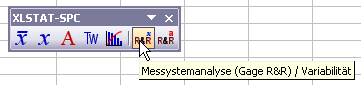The SPC Gage R&R dialog box will appear. Then select the data on the Excel sheet.

(Note: There are several ways of selecting data with XLSTAT - for further information, please check the section on selecting data.)

In this example, the data start from the first row, so it is quicker and easier to use column selection. That is why the letters corresponding to the columns are displayed in the selection boxes of the dialog box.

Select the column "Measurement" as Y / Measurements, and the column “Operator” as X / Operator, and the column "Part” as Parts. Choose the ANOVA method.

Activate the option Variable labels as the first row of the selections contains the variable names.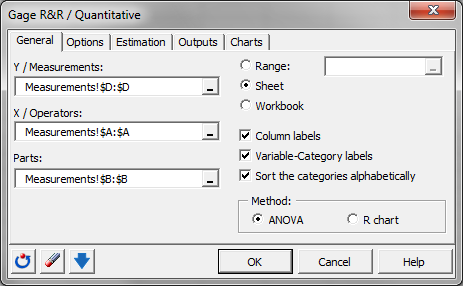In the Options tab, enter the value 6 as k sigma and choose the crossed ANOVA, in order to define the variance of the study as 6 times the global standard deviation of the sample.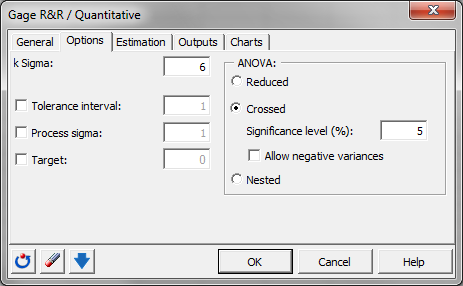In the Estimation tab, we chose the option R-bar.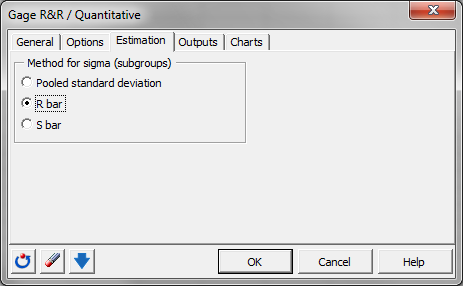In the Output tab, we activate all options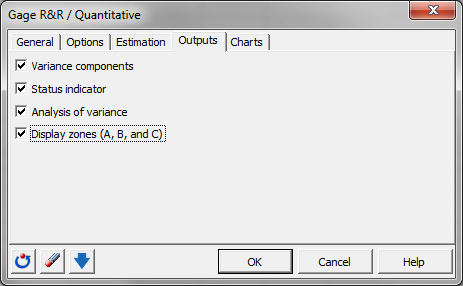In the Charts tab, we activate all options as well.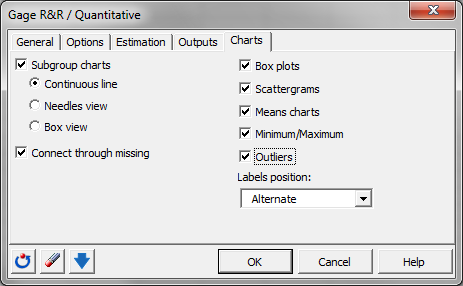The computations begin once you have clicked on OK. You are asked to confirm the number of rows and columns (this message can be bypassed by un-selecting the Ask for selections confirmation in the XLSTAT options panel).

## Interpreting the results of a Gage R&R quantitative analysis

The first results are the variance components together with its chart. You can see that the biggest part of the variance is caused by variation between parts (96.4% of the variance). The measurement system has a very low contribution to the total variance (3.6%) and it can therefore be qualified as capable.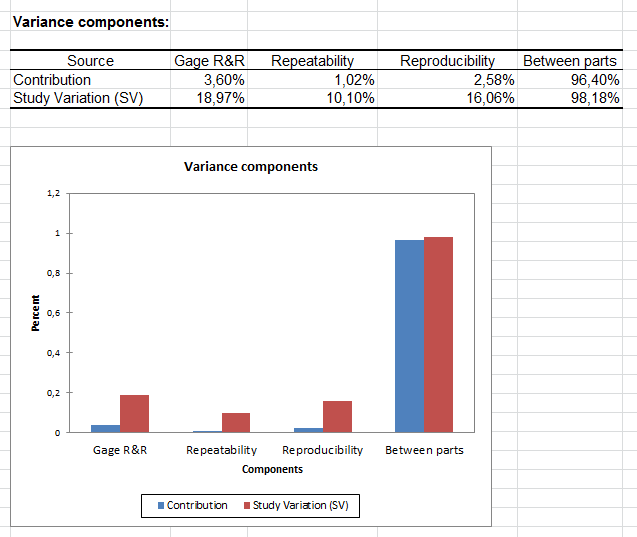The following tables give details on the distribution of the variance and the derived key indicators. The second table shows results for the study variation (based on the factor k Sigma set by the user to 6 in this example). More key indicators for the assessment of the measurement system are displayed in the third table.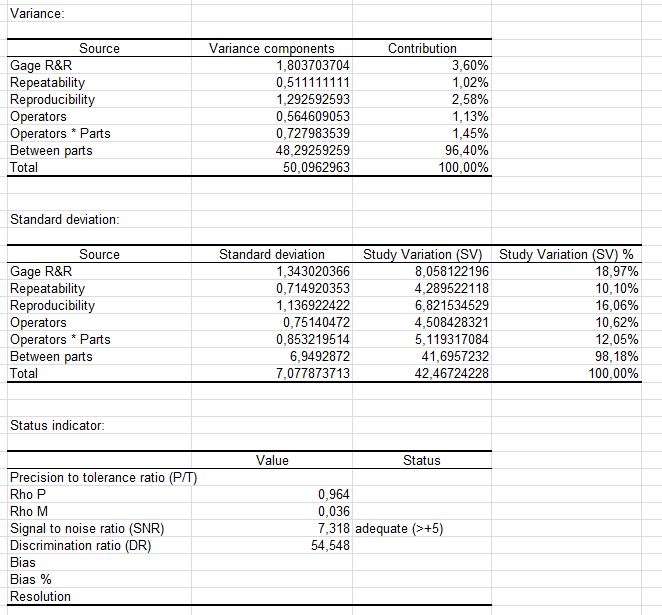If the ANOVA method has been selected like in this tutorial, then the results of the ANOVA with its GOF indicators and the table with the variance analysis are displayed. Look at the p-values to assess the significance level.The following tables are used to buld the X-bar control chart including the different control limits and central lines.

In the first table the data of the X-bar control chart starting with the group mean, group min and group max are displayed. After this the central line (CL), the lower (LCL) and the upper (UCL) control limit and the lower and upper zone limits for the A and B area are displayed for each group.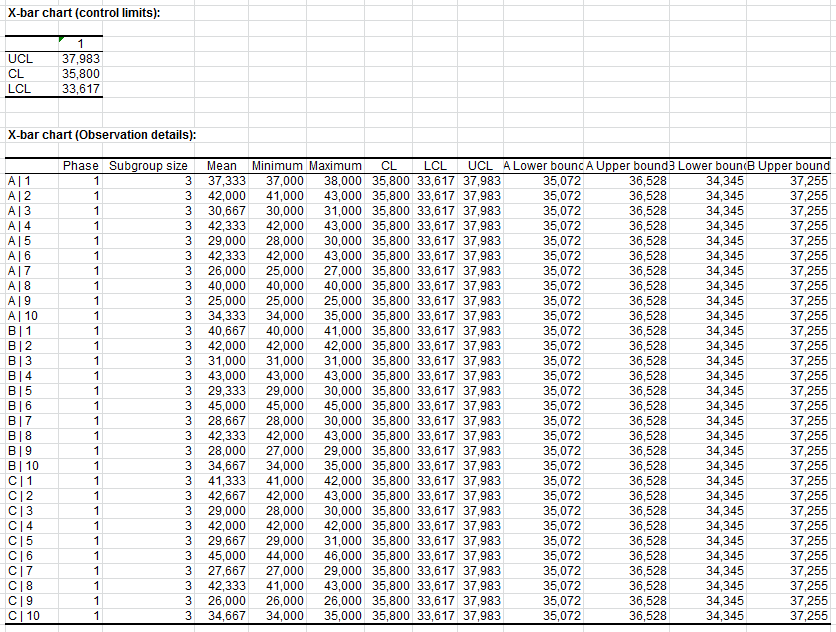Summing up you can discover all this in the X-bar control chart. The group means values are not between the lower and upper control limits. The variance between the parts is not under control.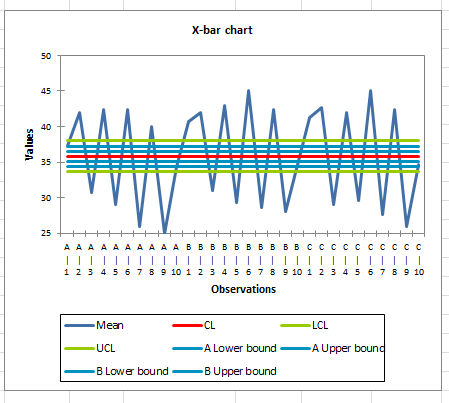Similar to the X-bar chart the data of the R chart are always inside the control limits and no special causes are present. This is a typical result of a measurement system analysis.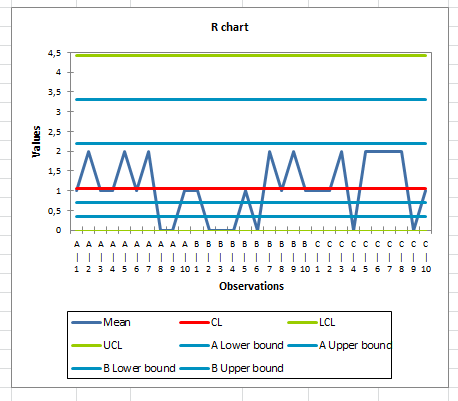Both control charts lets us come to the conclusion that the measurement system is capable.

The following charts show box plots and scatter grams for each operator and for each part. They allow comparing the different distributions visually.

We can see that the variation between the parts is important. The parts 2, 4, 6 and 8 have clearly higher measurement values than the parts 3, 5, 7 and 9.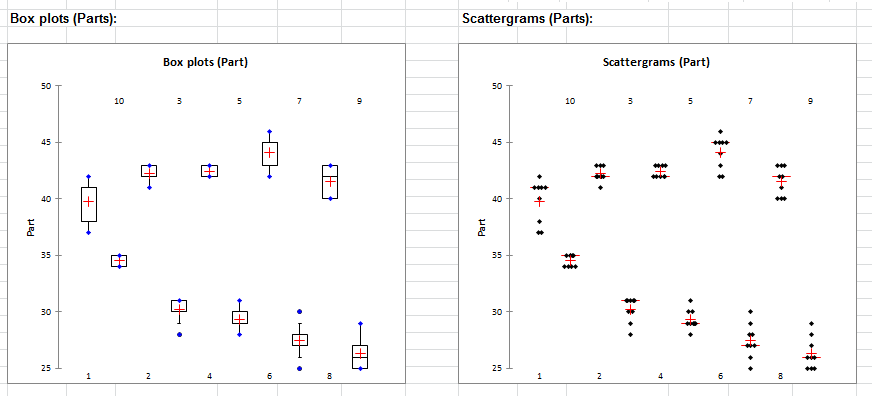The variance between the operators is very small. No differences are visible.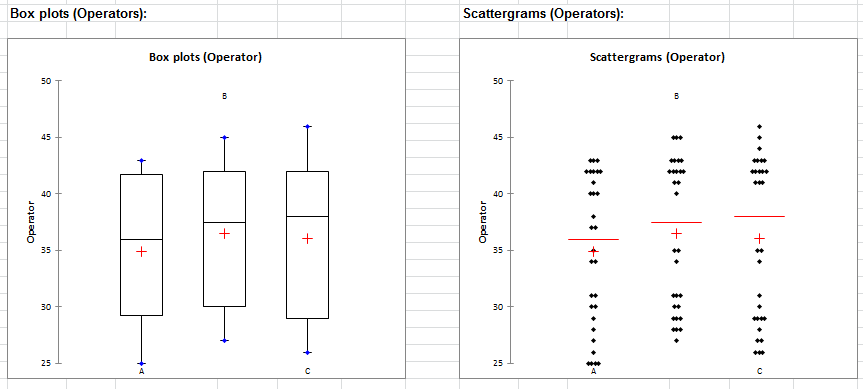Finally the mean value profiles for each part, for each operator and for each part*operator are displayed.

In the mean value chart for the parts, we find again the two different groups of parts.

In the mean value chart for the operators, we see differences, which are 10 times smaller than the variation between the parts.

In the two profile charts for the interaction part*operator we can see again that the profiles of the operators for each part are very close together. The chart of the profiles for the part by operator contains large variation between the different parts.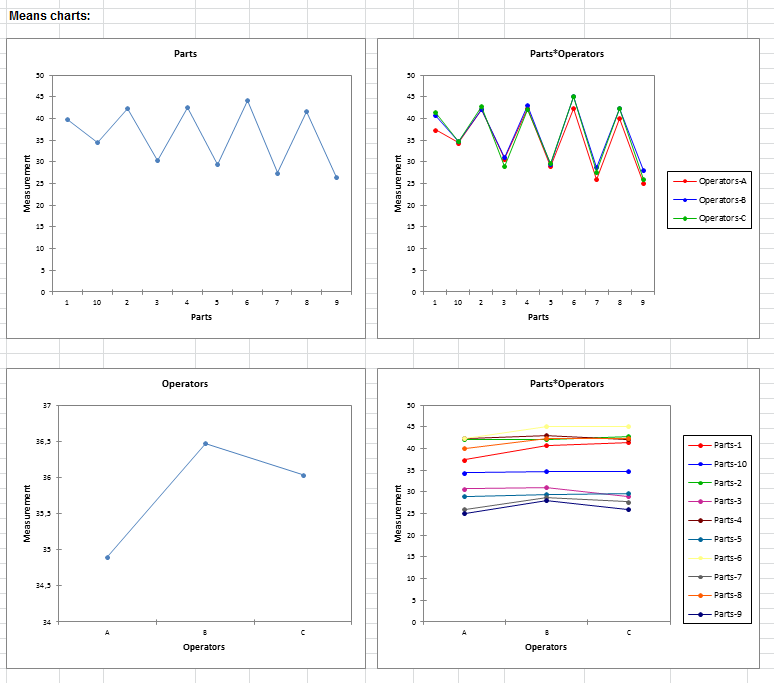### Contact Us

#### Contact our technical support team: support@xlstat.com

https://cdn.desk.com/
false
desk
Loading
seconds ago
a minute ago
minutes ago
an hour ago
hours ago
a day ago
days ago
about
false
Invalid characters found
/customer/portal/articles/autocomplete
9283